Next: Internal Diffusion in LJ Up: Applications to Lennard-Jones Clusters Previous: Applications to Lennard-Jones Clusters   Contents

##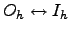Isomerisation of LJ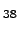We have applied the GT method to study the temperature dependence of the rate ofinterconversion of 38-atom Lennard-Jones cluster. The PES sample was taken from a previous study  and contained 1740 minima and 2072 transition states. Only geometrically distinct structures were considered when generating this sample because this way the dimensionality of the problem is reduced approximately by a factor of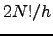, where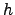is the order of the point group. Initial and final states in this sample roughly correspond to icosahedral-like and octahedral-like structures on the PES of this cluster. The assignment was made in Reference Wales02 by solving master equation numerically to find the eigenvector that corresponds to the smallest non-zero eigenvalue. As simple two-state dynamics are associated with exponential rise and decay of occupation probabilities there must exist a time scale on which all the exponential contributions to the solution of the master equation decay to zero except for the slowest . The sign of the components of the eigenvector corresponding to the slowest mode was used to classify the minima as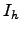or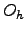in character .

The above sample was pruned to ensure that every minimum is reachable from any other minimum to create a digraph representation that contained 759 nodes including 43 source nodes and 5 sink nodes, and 2639 edges. The minimal, average and maximal degree for this graph were 2,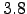and 84, respectively, and the graph density was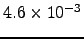. We have used the SDGT algorithm with the switching ratio set to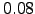to transform this graph for several values of temperature. In each of these calculations 622 out of 711 intermediate nodes were detached using SGT, and the remaining 89 intermediate nodes were detached using the GT algorithm optimised for dense graphs (DGT).

An Arrhenius plot depicting the dependence of the rate constant on temperature is shown in Figure 4.14 (a). The running time of SDGT algorithm was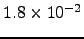seconds [this value was obtained by averaging over 10 runs and was the same for each SDGT run in Figure 4.14 (a)]. For comparison, the timings obtained using the SGT and DGT algorithms for the same problem were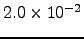and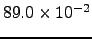seconds, respectively. None of the 43 total escape probabilities (one for every source) deviated from unity by more than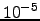for temperatures above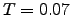(reduced units). For lower temperatures the probability was not conserved due to numerical imprecision.

The data obtained using SDGT method is compared with results from KMC simulation, which require increasingly more CPU time as the temperature is lowered. Figure 4.14 also shows the data for KMC simulations at temperatures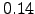,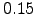,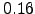,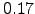and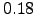. Each KMC simulation consisted of the generation of an ensemble of 1000 KMC trajectories from which the averages were computed. The cost of each KMC calculation is proportional to the average trajectory length, which is depicted in Figure 4.14 (b) as a function of the inverse temperature. The CPU timings for each of these calculations were (in the order of increasing temperature, averaged over five randomly seeded KMC simulations):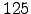,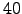,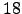,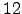, and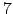seconds. It can be seen that using GT method we were able to obtain kinetic data for a wider range of temperatures and with less computational expense.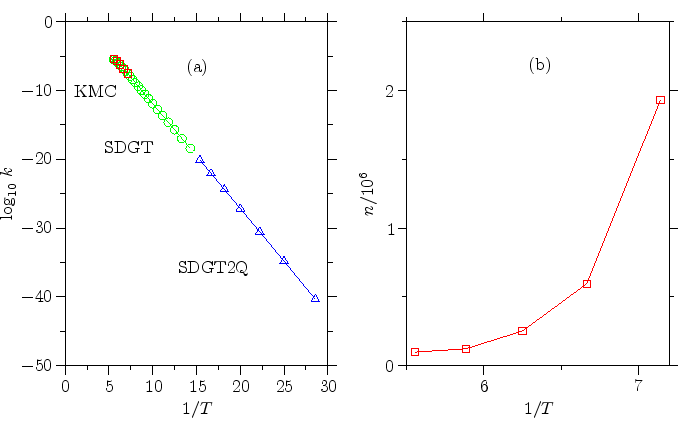Next: Internal Diffusion in LJ Up: Applications to Lennard-Jones Clusters Previous: Applications to Lennard-Jones Clusters   Contents
Semen A Trygubenko 2006-04-10